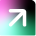# Momentum + Volatility InvestingOne of the promising factors in the emerging countries have been the momentum among the other factors as the growth is rampant and the developed market see it as a potential money multiplying opportunity. Same is the fundamental idea behind momentum investing, which proposes to invest in assets which have consistently been growing since a particular time frame. Here in this assignment the momentum factor have been used as the primary factor of investment and the volatility is been utilised to impact the weight of a stock in the portfolio. Including volatility has been quite the conservative practise as it helps in consistency in returns and to have an overall control on the max drawdown.

For momentum and volatility too there have been two time frames which they have been calculated viz. Momentum_longTerm, Momentum_shortTerm, Volatility_longTerm, Volatility_shortTerm. The long and short refers to a general time frame of 12 and 6 months respectively, stating that the data used for the calculation of the factor for a stock is of past 12 or 6 months. But since in this strategy we are using volatility as a weight determining parameter no such parameters were taken into consideration.

Firstly, the momentum indicator, which is built on the basis of momentum score, the white paper by the NSE India included this method of momentum calculation also used for the ETF NIFTY 200 Momentum 30.

Momentum Ratio = Price returns / Standard deviation price

For, 12 month momentum ratio,

12 months price returns = [P(M-1) / P(M-13)] – 1

Standard Deviation 12 = Annualised standard deviation of a stock’s logarithmic daily returns for the last one year. Similarly for the 6 months.

This momentum score can be used to filter, top stocks with maximum scores can be selected and then weighted accordingly.

But for the NSE index where a mixed score of both 6 months and 12 months is aggregated, a normalisation is done which is:

Zscore = (Momentum Ratio12 - Mean Momentum Ratios)/ Standard Deviation Momentum Ratios Where,

Mean Momentum Ratios = Mean of all the momentum ratios in case of NIFTY 200, the 200 stocks. Standard Deviation Momentum Ratios = Standard deviation of the momentum ratios of 200 stock Similarly for the 6 months.

The weighted average Zscore of the eligible stock would be:

Weighted average Zscore = [50% * (Zscore for 12 month’s momentum) + 50% * (Zscore for 6 month’s momentum)]

Normalised momentumscore = (1 + Weighted Avg. Zscore) : If weighted avg. Zscore >= 0 (1 - Weighted Avg. Zscore) : If weighted avg. Zscore < 0

For volatility in NIFTY low volatility 50 the volatility is calculated by standard deviation of the daily logarithmic price returns adjusted for any corporate actions. The weight also adjusted are in the inverse proportion to the volatility.

Methodology:

The aim is to surpass the benchmark of momentum strategy for all industry included having arguments for the parameters as follows:

Frequency: Monthly

Indicator: Momentum_longTerm

position sizing: inverse volatility

 Ann_returns Ann_risk Max_drawdown Sharpe ratio 20.60% 10.54% 21.37% 1.39

To accomplish this for each particular industry the results have to be extracted from the simulator, among the industries Diversified outstand in returns. (Quarterly, Momentum_shortTerm, Sector_cross sectional)

 Ann_returns Ann_risk Max_drawdown Sharpe ratio 40.14% 23.72% 29.28% 1.44

Since to diversify and focus on high returning sectors we included Automobiles and Textiles, producing results as follows: (Quarterly, Momentum_shortTerm, industry cross section, inverse_vol).

 Ann_returns Ann_risk Max_drawdown Sharpe ratio 32.86% 14.15% 28.65% 1.90

To further diversify we have constructed portfolio of by adding FMCG and Others sector (sector_count=5) and another portfolio including 7 sectors by adding banking and chemical sector to it, results as follows:

(Quarterly, momentum_shortTerm, sectional cross_sectional, 5 sector)

 Ann_returns Ann_risk Max_drawdown Sharpe ratio 27.32% 11.63% 23.86% 1.83

(Quarterly, momentum_shortTerm, sectional cross_sectional, 7 sectors)

 Ann_returns Ann_risk Max_drawdown Sharpe ratio 24.20% 10.65% 22.29% 1.71

Note that above results are excluding any kind of charges or tax deductions, also the frequency of any portfolio adjustment is quarterly which provides a trade-off between changes by the indicator and the transaction cost unlike the monthly or yearly frequency. Hence considering these factors an overall 2.5-5% deduction can be projected in te above results.

Future Scope:

Similar work on the momentum investing is done by Gary Anotonacci where he has described GEM (Global Equity Momentum), which included set of rules for multiple asset class along with equities. Based on GEM there were two strategies know as dual momentum and dual momentum plus. In this technique the absolute momentum was calculated which is one year momentum of the stock minus money momentum (EUR-USD rates in paper). The quantile whose momentum was negative was divested and the money divested remained in cash. For dual momentum plus the same technique but the cash left wash re-distributed among the positive quantiles. The study showed that the first (dual momentum) strategy reduced the fall of the portfolio whereas the second (dual momentum plus) showed an improved rebound after a fall in comparison to the momentum based investing.